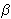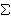# Simple Linear Regression

We live in a world that is filled with patterns – patterns all around us just waiting to be discovered. Some of these patterns are not as easily discovered because of the existence of outside noise.

Consider for example an experiment where a set of people were each given the task of drinking a number of beers and having their blood alcohol level taken afterwards. Some noise factors in this could include the height and weight of the individual, the types of drinks, the amount of food eaten, and the time between drinks. Even with this noise, though, we can still see a correlation between the number of drinks and their blood alcohol level. Consider the following graph showing people’s blood-alcohol level after a given number of drinks. The x-axis represents the number of drinks and the y-axis is the corresponding blood alcohol level.x 5 2 9 8 3 7 3 5 3 5 4 6 5 7 1 4 y 0.1 0.03 0.19 0.12 0.04 0.095 0.07 0.06 0.02 0.05 0.07 0.1 0.085 0.09 0.01 0.05

We can definitely see a correlation, and although the data doesn’t quite fit on a straight line. It leads us to ask further questions like can we use this data to build a model that estimates a person’s blood-alcohol level and how strong is this model?

One of the tools we can use to model this problem is linear regression. A linear regression takes a two-dimensional data set, with the assumption that one column (generally represented by the x variable) is independent and the second column (generally represented by the y variable) being dependent on the first column. The assumption is that the relationship between the two columns is linear and can be represented by the linear equation

y =0 +1x + e.

The right hand side of the above equation has three terms. The first two (0 and1) are the parameters of the linear equation (the y-intercept and slope respectively), while the third term of the right hand side of the above equation represents the error term. The error term represents the difference between this linear equation and the y values in the data provided. We are seeking a line that minimizes the error term. That is, we are seeking to minimize

D =i = 1 to n [yi – (0 +1xi)]2

There are several ways one could approach this problem. In fact, there are several lines that one could use to build a linear model. The first line that one may use to model these points is the one generated by only mean of the y values of the points, called the horizontal line regression.

For the data set above, the mean of the y values can be calculated as= 0.0738, so we could build a linear model based on this mean that would be y = 0.738. This horizontal line regression model is a horizontal line that predicts the same score (the mean), regardless of the x value. This lack of adjustments means it is generally a poor fit for most models. But as we will see later, this horizontal line regression model does serve a purpose in determining how well the model we develop performs.

A second attempt at solving this problem would be to generate the least squares line. This is the line that minimizes the D value listed above. We can see that D is a multi-variable polynomial, and we can find the minimum of such a polynomial using calculus, partial derivatives and Gaussian elimination (I will omit the work here because it deters us from the main point of this blog post, however Steven J. Miller has a good write-up of this).

The calculus leads us to the following equations:

SXY =i = 1 to n(xy) –
 (i = 1 to nx)(i = 1 to ny) n
SXX =i = 1 to n(x2) –
 (i = 1 to nx)2 n1 =
 SXY SXX0 =–1To calculate the least squares line for this example, we first need to calculate a few values:i = 1 to n(xy) = 6.98i = 1 to n(x2) = 443i = 1 to nx = 77i = 1 to ny = 1.18
Sxx = 72.44
Sxy = 1.30

This lets us evaluate that1 = 0.018
and0 = -0.0127

So the resulting linear equation for this data is= -0.0127 + 0.018*x

Below is a graph of the two attempts at building a linear model for this data.In the above image, the green line represents the horizontal line regression model and the blue line represents the least-squares line. As stated above, the horizontal line regression model is a horizontal line that does not adjust as the data changes. The least-squares line adjusts both the slope and y-intercept of this line according to the data provided to better fit the data provided. The question becomes how well does the least-squares line fit the data.

The Sum of Squares Error (SSE) sums the deviation at each point of our data from the least-squares line.

SSE =i = 1 to n(yii)2

A second metric that we are interested in is how well the horizontal line regression linear model estimates our data. This is called the Total Sum of Squares (SST).

SST =i = 1 to n(yi)2

The horizontal line regression model ignores the independent variable x from our data set and thus any line that takes this independent variable into account will be an improvement on the horizontal line regression model. Because of this, the SST sum is a worse case scenario of how poorly our model can perform.

Knowing now that SST is always greater than SSE, the regression sum of squares (SSR) is the difference between the total sum of squares and the sum of squares error.

SSR = SST – SSE

This tells us how much of the total sum of squares is accounted for by the model.

Finally, the coefficient of determination (r2) is defined by

r2 = SSR / SST

This tells SSR as a percentage of SST, or the amount of the variation in the data that is explained by the model.

So, check out my script on simple linear regression and let me know what you think.